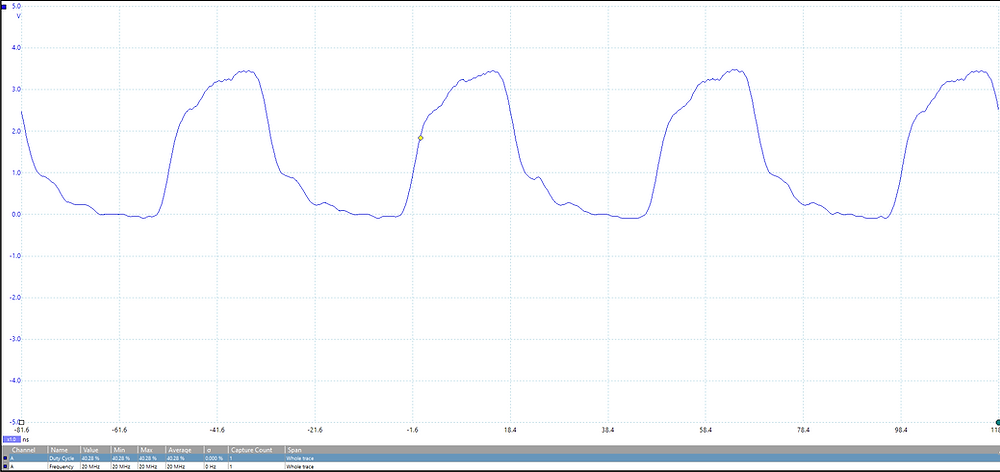Search

# MicroZed Chronicles: A Blast from the Past!

Modern FPGA devices really spoil us. They include PLLs, DCM, DSP etc. and a range of interfaces that significantly ease our developments. Recently though, I faced a situation where the PLL could not be used for safety reasons. This got me scratching my head a little about how I was going to generate a 20 MHz clock from a 50 MHz source without a PLL and also without changing the oscillator frequencies since the 50 MHz is supplied by another satellite payload in this case.Looking back in the notes I’ve gathered over the years, I remembered that the great Peter Alfke published several circuits that could be used in this situation to provide non-integer divisions and clock multiplication. These techniques often come in handy so I thought it would be good to refresh a couple of these techniques and show the performance in modern silicon (Basys 3 Artix-7 FPGA board).

Let’s start with looking at a simple clock doubler circuit. This circuit uses an XNOR gate to generate pulses for each edge of the input clock. As a delay is required to do this in one of the inputs of the XNOR gate, this is provided by the delay register. This logic design will of course not output a 50:50 duty cycle waveform.

By implementing this in a Basys 3 board and providing the clock to the circuit at 10 MHz, we would expect a 20 MHz output routed to the pins of the Pmod output pin. Careful selection of the drive strength, slew rate, pull down, and termination settings presented a reasonable waveform.In addition to the clock multiplication circuit, Peter also presented several division circuits, a divide by 1.5, 2.5, 3 and 5. All of these are based on the use of synchronous counters and then a latch circuit to generate the output.

All of these can be implemented easily in VHDL or Verilog. For the following examples, I used a 10 MHz clock to demonstrate the division algorithms. The first implementation is a divide by 1.5, followed by a divide by 2.5, 3 and 5. The division by 3 and 5 also provide a 50:50 duty cycle.

We should expect to see frequencies like the following when working with a 10 MHz input clock:The RTL for the multiplier and counter as implemented is shown below.

library IEEE;

use IEEE.STD_LOGIC_1164.ALL;

use IEEE.NUMERIC_STD.ALL;

entity top is port(

clk : in std_logic;

clk_1d5 : out std_logic;

clk_2d5 : out std_logic;

clk_3 : out std_logic;

clk_5 : out std_logic;

clk_op : out std_logic

);

end top;

architecture Behavioral of top is

signal inv : std_logic := '0';

signal delay : std_logic := '0';

signal clk_double : std_logic :='0';

signal clk_2d5_cnt : unsigned(2 downto 0) := (others => '0');

signal clk_1d5_cnt : unsigned(1 downto 0) := (others => '0');

signal clk_3_cnt : unsigned(1 downto 0) := (others => '0');

signal clk_5_cnt : unsigned(2 downto 0) := (others => '0');

begin

clk_delay : process(clk_double)

begin

if rising_edge(clk_double) then

delay <= inv;

end if;

end process;

inv <= not delay;

clk_double <= clk XNOR inv;

clk_op <= clk_double;

delay_1_5 : process(clk)

begin

if rising_edge(clk) then

if clk_1d5_cnt = 2 then

clk_1d5_cnt <= (others => '0');

else

clk_1d5_cnt <= clk_1d5_cnt + 1;

end if;

end if;

end process;

clk_1d5 <= '1' when clk_1d5_cnt = 0 and clk ='1' else

'1' when clk_1d5_cnt = 1 and clk ='1' else

'1' when clk_1d5_cnt = 1 and clk ='0' else

'1' when clk_1d5_cnt = 2 and clk ='0' else

'0';

delay_2_5 : process(clk)

begin

if rising_edge(clk) then

if clk_2d5_cnt = 4 then

clk_2d5_cnt <= (others => '0');

else

clk_2d5_cnt <= clk_2d5_cnt + 1;

end if;

end if;

end process;

clk_2d5 <= '1' when clk_2d5_cnt = 1 and clk ='1' else

'1' when clk_2d5_cnt = 1 and clk ='0' else

'1' when clk_2d5_cnt = 3 and clk ='0' else

'1' when clk_2d5_cnt = 4 and clk ='1' else

'0';

delay_3 : process(clk)

begin

if rising_edge(clk) then

if clk_3_cnt = 2 then

clk_3_cnt <= (others => '0');

else

clk_3_cnt <= clk_3_cnt + 1;

end if;

end if;

end process;

clk_3 <= '1' when clk_3_cnt = 1 and clk ='1' else

'1' when clk_3_cnt = 1 and clk ='0' else

'1' when clk_3_cnt = 2 and clk ='1' else

'0';

delay_5 : process(clk)

begin

if rising_edge(clk) then

if clk_5_cnt = 4 then

clk_5_cnt <= (others => '0');

else

clk_5_cnt <= clk_5_cnt + 1;

end if;

end if;

end process;

clk_5 <= '1' when clk_5_cnt = 0 and clk ='1' else

'1' when clk_5_cnt = 0 and clk ='0' else

'1' when clk_5_cnt = 1 and clk ='1' else

'1' when clk_5_cnt = 1 and clk ='0' else

'1' when clk_5_cnt = 2 and clk ='1' else

'0';

end Behavioral;

Running the algorithm on the Basys 3 hardware and observing the output with the scope shows the following waveforms.Division by 1.5 to generate a 6.66MHz clockDivision by 2.5 to generate a 4 MHz clockDivision by 3 to generate a 3.33 MHz clockDivision by 5 to generate a 2 MHz clock

These circuits work well. Of course, there will be some drift with temperature and voltage changes, especially in the multiplier circuit.

Of course, PLL or DCM should be your first choice for implementing clock multiplication and division if available.

The techniques that Peter demonstrated so well in his tech notes that helped so many developers in the earlier days of FPGA development may come in handy for some applications. Such elegant techniques deserve remembering from time to time.